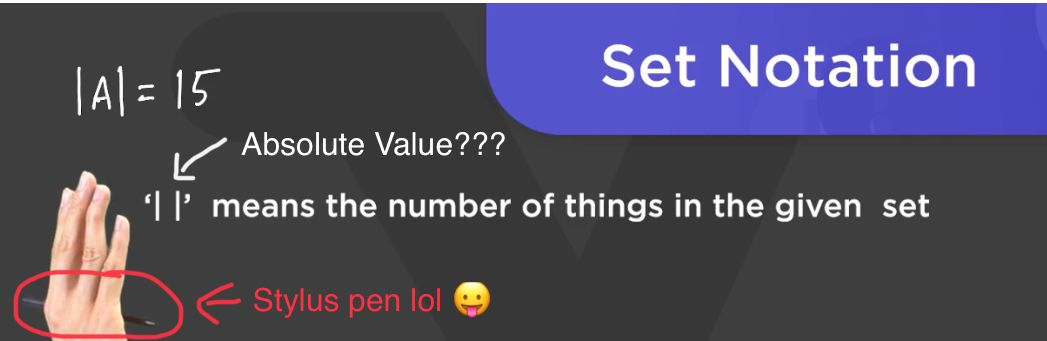# || symbol (+Stylus pen)

•The “| |” I just learnt means “Set” but it looks the same as another symbol that means “Absolute value”. Are there any connections? Why did mathematicians choose a same pair of symbols to mean 2 things?
Also Prof Loh was holding his stylus penwhen he was pointing towards the |A|• I don't know, but maybe for the same reason that ∆=discriminant=change/difference=triangle shape? (That reason is, mathematicians felt like making life harder for us. (Or because they ran out of symbols to use.))

• @RZ923Whenever you see the absolute value symbol written next to the name of a set, it refers to the number
of elements in that set. It doesn't technically mean the set itself, but this measure of how large the set is.

$$\text{ Example: } A = \{2, 3, 5, 7, 11\}$$

$$|A| = 5$$

$$\text{ Example: } B = \{2, 4, 6, 8\}$$

$$|A| = 4$$

If you write out $$A$$ with no vertical bars, then it refers to the set with the elements listed. It's similar to how when I refer to a person's name, like @RZ923, I'm referring to the person, not some numerical characteristic like age or Mock AMC 8 test scoreor anything else.

Let's look at the comparison between the set name with the vertical bars and the set name without the vertical bars:

If I were to write the PIE formula for sets $$A$$ and $$B,$$ but I didn't use the vertical bars, then what would that mean?

For example:

$$A \cup B = A + B - A \cap B$$

Technically, this really means the following:

$$\{2, 3, 4, 5, 6, 7, 8, 11\} = \{2, 3, 5, 7, 11\} + \{2, 4, 6, 8\} - \{2\}$$

See the difference when you add the vertical bars?\begin{aligned} \textcolor{red}{ |A \cup B|} &= \text{ } \text{ } \text{ } \text{ } \text{ } \text{ } \text{ } \text{ } \text{ } \text{ } \text{ } \textcolor{blue}{|A|} \text{ } \text{ } \text{ } \text{ } \text{ } \text{ } \text{ } \text{ } \text{ } \text{ } \text{ } +\text{ } \text{ } \text{ } \text{ } \text{ } \text{ } \text{ } \text{ } \text{ } \text{ } \text{ } \text{ }\textcolor{purple}{|B|} \text{ } \text{ } \text{ } \text{ } \text{ } \text{ } \text{ } \text{ } \text{ } \text{ } \text{ }- \text{ } \text{ } \text{ } \text{ } \text{ } \text{ } \text{ } \text{ } \text{ }\textcolor{green}{|A \cap B|} \\ \textcolor{red}{\#\text{ elements in } A \cup B } &= \textcolor{blue}{\# \text{ elements in } A} + \textcolor{purple}{\# \text{ elements in } B} - \textcolor{green}{\# \text{ elements in } A \cap B} \\ \textcolor{red}{8} \text{ } \text{ } \text{ } \text{ } \text{ } &= \text{ } \text{ } \text{ } \text{ } \text{ } \text{ } \text{ } \text{ } \text{ } \text{ } \text{ } \text{ } \text{ }\textcolor{blue}{5} \text{ } \text{ } \text{ } \text{ } \text{ } \text{ } \text{ } \text{ } \text{ } \text{ } \text{ } \text{ }+ \text{ } \text{ } \text{ } \text{ } \text{ } \text{ } \text{ } \text{ } \text{ } \text{ } \text{ } \text{ } \text{ } \text{ }\textcolor{purple}{4} \text{ } \text{ } \text{ } \text{ } \text{ } \text{ } \text{ } \text{ } \text{ } \text{ } \text{ } \text{ } \text{ }- \text{ } \text{ } \text{ } \text{ } \text{ } \text{ } \text{ } \text{ } \text{ } \text{ } \text{ } \text{ } \text{ } \text{ } \text{ }\textcolor{green}{1} \\ \end{aligned}

$$\text{ Set A } \text{ } \text{ } \text{ } \text{ } \text{ } \text{ } \text{ } \text{ } \text{ } \text{ } \text{ } \text{ } \text{ } \text{ } \text{ } \text{ } \text{ } \text{ } \text{ } \text{ } \text{ } \text{ } \text{ } \text{ Set B } \text{ } \text{ } \text{ } \text{ } \text{ } \text{ } \text{ } \text{ } \text{ } \text{ } \text{ } \text{ } \text{ } \text{ } \text{ } \text{ } \text{ } \text{ } \text{ } A \cap B \text{ } \text{ } \text{ } \text{ } \text{ } \text{ } \text{ } \text{ } \text{ } \text{ } \text{ } \text{ } \text{ } \text{ } \text{ } \text{ } \text{ } \text{ } \text{ } \text{ } \text{ } \text{ } A \cup B \text{ } \text{ } \text{ } \text{ } \text{ } \text{ } \text{ } \text{ } \text{ } \text{ } \text{ } \text{ }$$We usually use the vertical bars along with the set names when we're using PIE because we are trying to figure out how many ways or elements there are.Theagrees!